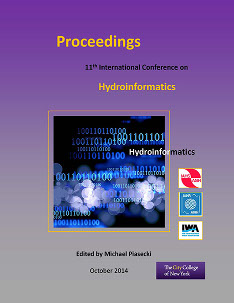## International Conference on Hydroinformatics

Presentation

8-1-2014

#### Abstract

Iran, has caused most of the water used and as much as possible to avoid losses. One of the important parameters in agriculture is water distribution uniformity coefficient (CU) in sprinkler irrigation. CU amount of water sprinkler operating depends on different pressure heads (P), riser height (RH), distance between sprinklers on lateral pipes (Sl) and the distance between lateral pipes (Sm). The best combination of the above parameters for maximum CU, is still unknown for applicators. In this research, CU quantities of zb model sprinkler (made in Iran) were measured at Hashemabad cotton research station of Gorgan under 3 different pressure heads (2.5, 3 and 3.5 atm), 2 riser heads (60 and 100 cm) and 7 sprinkler (Sl×Sm including 9×12, 9×15, 12×12, 15×12, 12×18, 15×15, 15×18m) arrangements. By using differential evolution algorithm (DE), CU equation was optimized and the best optimized coefficients obtained. In this algorithm, the coefficients F and CR equal to 2 and 0.5, respectively, with a population of 100 members and 1000 number of generations (iterations), provides the best results. Absolute error between the results of this algorithm with the measured results is 2.2%. As well as values Wilmot (d) and the root-mean square error (RMSE), equal to 0.919 and 2.126, respectively. This results show that this algorithm has high accuracy to estimate water distribution uniformity.